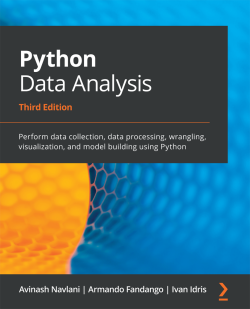### Python Data Analysis - Third Edition4 (1 reviews total)
By Avinash Navlani , Armando Fandango , Ivan Idris
Advance your knowledge in tech with a Packt subscription

• Instant online access to over 7,500+ books and videos
• Constantly updated with 100+ new titles each month
• Breadth and depth in over 1,000+ technologies

#### About this book

Data analysis enables you to generate value from small and big data by discovering new patterns and trends, and Python is one of the most popular tools for analyzing a wide variety of data. With this book, you’ll get up and running using Python for data analysis by exploring the different phases and methodologies used in data analysis and learning how to use modern libraries from the Python ecosystem to create efficient data pipelines.

Starting with the essential statistical and data analysis fundamentals using Python, you’ll perform complex data analysis and modeling, data manipulation, data cleaning, and data visualization using easy-to-follow examples. You’ll then understand how to conduct time series analysis and signal processing using ARMA models. As you advance, you’ll get to grips with smart processing and data analytics using machine learning algorithms such as regression, classification, Principal Component Analysis (PCA), and clustering. In the concluding chapters, you’ll work on real-world examples to analyze textual and image data using natural language processing (NLP) and image analytics techniques, respectively. Finally, the book will demonstrate parallel computing using Dask.

By the end of this data analysis book, you’ll be equipped with the skills you need to prepare data for analysis and create meaningful data visualizations for forecasting values from data.

Publication date:
February 2021
Publisher
Packt
Pages
478
ISBN
9781789955248

NumPy and pandas

Now that we have understood data analysis, its process, and its installation on different platforms, it's time to learn about NumPy arrays and pandas DataFrames. This chapter acquaints you with the fundamentals of NumPy arrays and pandas DataFrames. By the end of this chapter, you will have a basic understanding of NumPy arrays, and pandas DataFrames and their related functions.

pandas is named after panel data (an econometric term) and Python data analysis and is a popular open-source Python library. We shall learn about basic pandas functionalities, data structures, and operations in this chapter. The official pandas documentation insists on naming the project pandas in all lowercase letters. The other convention the pandas project insists on is the import pandas as pd import statement.

In this chapter, our focus will be on the following topics:

• Understanding...

# Technical requirements

This chapter has the following technical requirements:

# Understanding NumPy arrays

NumPy can be installed on a PC using pip or brew but if the user is using the Jupyter Notebook, then there is no need to install it. NumPy is already installed in the Jupyter Notebook. I will suggest to you to please use the Jupyter Notebook as your IDE because we are executing all the code in the Jupyter Notebook. We have already shown in Chapter 1, Getting Started with Python Libraries, how to install Anaconda, which is a complete suite for data analysis. NumPy arrays are a series of homogenous items. Homogenous means the array will have all the elements of the same data type. Let's create an array using NumPy. You can create an array using the array() function with a list of items. Users can also fix the data type of an array. Possible data types are bool, int, float, long, double, and long double.

Let's see how to create an empty array:

`# Creating an arrayimport numpy as npa = np.array([2,4,6,8,10])print(a)Output:[ 2 4 6 8 10]`

Another way to create...

# Array features

In general, NumPy arrays are a homogeneous kind of data structure that has the same types of items. The main benefit of an array is its certainty of storage size because of its same type of items. A Python list uses a loop to iterate the elements and perform operations on them. Another benefit of NumPy arrays is to offer vectorized operations instead of iterating each item and performing operations on it. NumPy arrays are indexed just like a Python list and start from 0. NumPy uses an optimized C API for the fast processing of the array operations.

Let's make an array using the arange() function, as we did in the previous section, and let's check its data type:

`# Creating an array using arange()import numpy as npa = np.arange(1,11)print(type(a))print(a.dtype)Output:<class 'numpy.ndarray'>int64`

When you use type(), it returns numpy.ndarray. This means that the type() function returns the type of the container. When you use dtype(), it will return...

# Selecting array elements

In this section, we will see how to select the elements of the array. Let's see an example of a 2*2 matrix:

`a = np.array([[5,6],[7,8]])print(a)Output:[[5 6] [7 8]]`

In the preceding example, the matrix is created using the array() function with the input list of lists.

Selecting array elements is pretty simple. We just need to specify the index of the matrix as a[m,n]. Here, m is the row index and n is the column index of the matrix. We will now select each item of the matrix one by one as shown in the following code:

`print(a[0,0])Output: 5print(a[0,1])Output: 6printa([1,0])Output: 7printa([1,1])Output: 8`

In the preceding code sample, we have tried to access each element of an array using array indices. You can also understand this by the diagram mentioned here: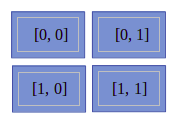In the preceding diagram, we can see it has four blocks and each block represents the element of an array. The values written in each block show its indices.

In this section, we have understood the...

# NumPy array numerical data types

Python offers three types of numerical data types: integer type, float type, and complex type. In practice, we need more data types for scientific computing operations with precision, range, and size. NumPy offers a bulk of data types with mathematical types and numbers. Let's see the following table of NumPy numerical types:

 Data Type Details bool This is a Boolean type that stores a bit and takes True or False values. inti Platform integers can be either int32 or int64. int8 Byte store values range from -128 to 127. int16 This stores integers ranging from -32768 to 32767. int32 This stores integers ranging from -2 ** 31 to 2 ** 31 -1. int64 This stores integers ranging from -2 ** 63 to 2 ** 63 -1. uint8 This stores unsigned integers ranging from 0 to 255. uint16 This stores unsigned integers ranging from 0 to 65535. uint32 This stores unsigned integers ranging from 0 to 2 ** 32 –...

# dtype objects

We have seen in earlier sections of the chapter that dtype tells us the type of individual elements of an array. NumPy array elements have the same data type, which means that all elements have the same dtype. dtype objects are instances of the numpy.dtype class:

`# Creating an arrayimport numpy as npa = np.array([2,4,6,8,10])print(a.dtype)Output: 'int64'`

dtype objects also tell us the size of the data type in bytes using the itemsize property:

`print(a.dtype.itemsize)Output:8`

# Data type character codes

Character codes are included for backward compatibility with Numeric. Numeric is the predecessor of NumPy. Its use is not recommended, but the code is supplied here because it pops up in various locations. You should use the dtype object instead. The following table lists several different data types and the character codes related to them:

 Type Character Code Integer i Unsigned integer u Single-precision float f Double-precision float d Bool b Complex D String S Unicode U Void V

Let's take a look at the following code to produce an array of single-precision floats:

`# Create numpy array using arange() functionvar1=np.arange(1,11, dtype='f')print(var1)Output:[ 1.,  2.,  3.,  4.,  5.,  6.,  7.,  8.,  9., 10.]`

Likewise, the following code creates an array of complex numbers:

`print(np.arange(1,6, dtype='D'))Output:[1.+0.j, 2.+0.j, 3.+0.j, 4.+0.j, 5.+0.j]`

# dtype constructors

There are lots of ways to create data types using constructors. Constructors are used to instantiate or assign a value to an object. In this section, we will understand data type creation with the help of a floating-point data example:

• To try out a general Python float, use the following:
`print(np.dtype(float))Output: float64`
• To try out a single-precision float with a character code, use the following:
`print(np.dtype('f'))Output: float32`
• To try out a double-precision float with a character code, use the following:
`print(np.dtype('d'))Output: float64`
• To try out a dtype constructor with a two-character code, use the following:
`print(np.dtype('f8'))Output: float64`

Here, the first character stands for the type and a second character is a number specifying the number of bytes in the type, for example, 2, 4, or 8.

# dtype attributes

The dtype class offers several useful attributes. For example, we can get information about the character code of a data type using the dtype attribute:

`# Create numpy array var2=np.array([1,2,3],dtype='float64')print(var2.dtype.char)Output: 'd'`

The type attribute corresponds to the type of object of the array elements:

`print(var2.dtype.type)Output: <class 'numpy.float64'>`

Now that we know all about the various data types used in NumPy arrays, let's start manipulating them in the next section.

# Manipulating array shapes

In this section, our main focus is on array manipulation. Let's learn some new Python functions of NumPy, such as reshape(), flatten(), ravel(), transpose(), and resize():

• reshape() will change the shape of the array:
`# Create an arrayarr = np.arange(12)print(arr)Output: [ 0  1  2  3  4  5  6  7  8  9 10 11]# Reshape the array dimensionnew_arr=arr.reshape(4,3)print(new_arr)Output: [[ 0,  1,  2],               [ 3,  4,  5],               [ 6,  7,  8],               [ 9, 10, 11]]# Reshape the array dimensionnew_arr2=arr.reshape(3,4)print(new_arr2)Output:array([[ 0,  1,  2,  3],       [ 4,  5,  6,  7],       [ 8,  9, 10, 11]])`
• Another operation that can be applied to arrays is flatten(). flatten() transforms an n-dimensional array into a one-dimensional array:
`# Create an arrayarr=np.arange(1,10).reshape(3,3)print(arr)Output: [[1 2 3] [4 5 6] [7 8 9]]print(arr.flatten())Output: [1 2 3 4 5 6 7 8 9]`
• The ravel() function is similar to the flatten() function...

# The stacking of NumPy arrays

NumPy offers a stack of arrays. Stacking means joining the same dimensional arrays along with a new axis. Stacking can be done horizontally, vertically, column-wise, row-wise, or depth-wise:

• Horizontal stacking: In horizontal stacking, the same dimensional arrays are joined along with a horizontal axis using the hstack() and concatenate() functions. Let's see the following example:
`arr1 = np.arange(1,10).reshape(3,3)print(arr1)Output:[[1 2 3] [4 5 6] [7 8 9]]`

We have created one 3*3 array; it's time to create another 3*3 array:

`arr2 = 2*arr1print(arr2)Output:[[ 2 4 6] [ 8 10 12] [14 16 18]]`

After creating two arrays, we will perform horizontal stacking:

`# Horizontal Stackingarr3=np.hstack((arr1, arr2))print(arr3)Output:[[ 1 2 3 2 4 6] [ 4 5 6 8 10 12] [ 7 8 9 14 16 18]]`

In the preceding code, two arrays are stacked horizontally along the x axis. The concatenate() function can also be used to generate the horizontal stacking with axis parameter...

# Partitioning NumPy arrays

NumPy arrays can be partitioned into multiple sub-arrays. NumPy offers three types of split functionality: vertical, horizontal, and depth-wise. All the split functions by default split into the same size arrays but we can also specify the split location. Let's look at each of the functions in detail:

• Horizontal splitting: In horizontal split, the given array is divided into N equal sub-arrays along the horizontal axis using the hsplit() function. Let's see how to split an array:
`# Create an arrayarr=np.arange(1,10).reshape(3,3)print(arr)Output: [[1 2 3] [4 5 6] [7 8 9]]# Peroform horizontal splittingarr_hor_split=np.hsplit(arr, 3)print(arr_hor_split)Output:[array([,       ,       ]), array([,       ,       ]), array([,       ,       ])]`

In the preceding code, the hsplit(arr, 3) function divides the array into three sub-arrays. Each part is a column of the original array.

• Vertical splitting: In vertical split, the given...

# Changing the data type of NumPy arrays

As we have seen in the preceding sections, NumPy supports multiple data types, such as int, float, and complex numbers. The astype() function converts the data type of the array. Let's see an example of the astype() function:

`# Create an arrayarr=np.arange(1,10).reshape(3,3)print("Integer Array:",arr)# Change datatype of arrayarr=arr.astype(float)# print arrayprint("Float Array:", arr)# Check new data type of arrayprint("Changed Datatype:", arr.dtype)`

In the preceding code, we have created one NumPy array and checked its data type using the dtype attribute.

Let's change the data type of an array using the astype() function:

`# Change datatype of arrayarr=arr.astype(float)# Check new data type of arrayprint(arr.dtype)Output:float64`

In the preceding code, we have changed the column data type from integer to float using astype().

The tolist() function converts a NumPy array into a Python list. Let's see an...

# Creating NumPy views and copies

Some of the Python functions return either a copy or a view of the input array. A Python copy stores the array in another location while a view uses the same memory content. This means copies are separate objects and treated as a deep copy in Python. Views are the original base array and are treated as a shallow copy. Here are some properties of copies and views:

• Modifications in a view affect the original data whereas modifications in a copy do not affect the original array.
• Views use the concept of shared memory.
• Copies require extra space compared to views.
• Copies are slower than views.

Let's understand the concept of copy and view using the following example:

`# Create NumPy Arrayarr = np.arange(1,5).reshape(2,2)print(arr)Output:[[1, 2],[3, 4]]`

After creating a NumPy array, let's perform object copy operations:

`# Create no copy only assignmentarr_no_copy=arr# Create Deep Copyarr_copy=arr.copy()# Create shallow copy using Viewarr_view=arr...`

# Slicing NumPy arrays

Slicing in NumPy is similar to Python lists. Indexing prefers to select a single value while slicing is used to select multiple values from an array.

NumPy arrays also support negative indexing and slicing. Here, the negative sign indicates the opposite direction and indexing starts from the right-hand side with a starting value of -1: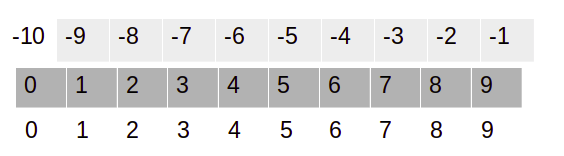Let's check this out using the following code:

`# Create NumPy Arrayarr = np.arange(0,10)print(arr)Output: [0, 1, 2, 3, 4, 5, 6, 7, 8, 9]`

In the slice operation, we use the colon symbol to select the collection of values. Slicing takes three values: start, stop, and step:

`print(arr[3:6])Output: [3, 4, 5]`

This can be represented as follows: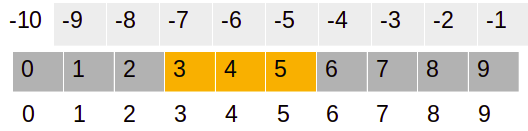In the preceding example, we have used 3 as the starting index and 6 as the stopping index:

`print(arr[3:])Output: array([3, 4, 5, 6, 7, 8, 9])`

In the preceding example, only the starting index is given. 3 is the starting index. This slice operation will select the values from the starting index...

# Boolean and fancy indexing

Indexing techniques help us to select and filter elements from a NumPy array. In this section, we will focus on Boolean and fancy indexing. Boolean indexing uses a Boolean expression in the place of indexes (in square brackets) to filter the NumPy array. This indexing returns elements that have a true value for the Boolean expression:

`# Create NumPy Arrayarr = np.arange(21,41,2)print("Orignial Array:\n",arr)# Boolean Indexingprint("After Boolean Condition:",arr[arr>30])Output:Orignial Array: [21 23 25 27 29 31 33 35 37 39]After Boolean Condition: [31 33 35 37 39]`

Fancy indexing is a special type of indexing in which elements of an array are selected by an array of indices. This means we pass the array of indices in brackets. Fancy indexing also supports multi-dimensional arrays. This will help us to easily select and modify a complex multi-dimensional set of arrays. Let's see an example as follows to understand fancy indexing:

`# Create...`

# Broadcasting arrays

Python lists do not support direct vectorizing arithmetic operations. NumPy offers a faster-vectorized array operation compared to Python list loop-based operations. Here, all the looping operations are performed in C instead of Python, which makes it faster. Broadcasting functionality checks a set of rules for applying binary functions, such as addition, subtraction, and multiplication, on different shapes of an array.

Let's see an example of broadcasting:

`# Create NumPy Arrayarr1 = np.arange(1,5).reshape(2,2) print(arr1)Output:[[1 2] [3 4]]# Create another NumPy Arrayarr2 = np.arange(5,9).reshape(2,2) print(arr2)Output:[[5 6] [7 8]]# Add two matricesprint(arr1+arr2)Output:[[ 6 8] [10 12]]`

In all three preceding examples, we can see the addition of two arrays of the same size. This concept is known as broadcasting:

`# Multiply two matricesprint(arr1*arr2)Output:[[ 5 12] [21 32]]`

In the preceding example, two matrices were multiplied. Let's perform addition...

# Creating pandas DataFrames

The pandas library is designed to work with a panel or tabular data. pandas is a fast, highly efficient, and productive tool for manipulating and analyzing string, numeric, datetime, and time-series data. pandas provides data structures such as DataFrames and Series. A pandas DataFrame is a tabular, two-dimensional labeled and indexed data structure with a grid of rows and columns. Its columns are heterogeneous types. It has the capability to work with different types of objects, carry out grouping and joining operations, handle missing values, create pivot tables, and deal with dates. A pandas DataFrame can be created in multiple ways. Let's create an empty DataFrame:

`# Import pandas libraryimport pandas as pd# Create empty DataFramedf = pd.DataFrame()# Header of dataframe. df.head()Output:_ `

In the preceding example, we have created an empty DataFrame. Let's create a DataFrame using a dictionary of the list:

`# Create dictionary of listdata = {&apos...`

# Understanding pandas Series

pandas Series is a one-dimensional sequential data structure that is able to handle any type of data, such as string, numeric, datetime, Python lists, and dictionaries with labels and indexes. Series is one of the columns of a DataFrame. We can create a Series using a Python dictionary, NumPy array, and scalar value. We will also see the pandas Series features and properties in the latter part of the section. Let's create some Python Series:

• Using a Python dictionary: Create a dictionary object and pass it to the Series object. Let's see the following example:
`# Creating Pandas Series using Dictionarydict1 = {0 : 'Ajay', 1 : 'Jay', 2 : 'Vijay'}# Create Pandas Seriesseries = pd.Series(dict1)# Show seriesseriesOutput:0     Ajay1      Jay2    Vijaydtype: object`
• Using a NumPy array: Create a NumPy array object and pass it to the Series object. Let's see the following example:
`#Load Pandas and NumPy libraries...`

# Reading and querying the Quandl data

In the last section, we saw pandas DataFrames that have a tabular structure similar to relational databases. They offer similar query operations on DataFrames. In this section, we will focus on Quandl. Quandl is a Canada-based company that offers commercial and alternative financial data for investment data analyst. Quandl understands the need for investment and financial quantitative analysts. It provides data using API, R, Python, or Excel.

In this section, we will retrieve the Sunspot dataset from Quandl. We can use either an API or download the data manually in CSV format.

Let's first install the Quandl package using pip:

`\$ pip3 install Quandl`

If you want to install the API, you can do so by downloading installers from https://pypi.python.org/pypi/Quandl or by running the preceding command.

Using the API is free, but is limited to 50 API calls per day. If you require more API calls, you will have to request an authentication key. The code...

# Describing pandas DataFrames

The pandas DataFrame has a dozen statistical methods. The following table lists these methods, along with a short description of each:

 Method Description describes This method returns a small table with descriptive statistics. count This method returns the number of non-NaN items. mad This method calculates the mean absolute deviation, which is a robust measure similar to standard deviation. median This method returns the median. This is equivalent to the value at the 50th percentile. min This method returns the minimum value. max This method returns the maximum value. mode This method returns the mode, which is the most frequently occurring value. std This method returns the standard deviation, which measures dispersion. It is the square root of the variance. var This method returns the variance. skew This method returns skewness. Skewness is indicative of the distribution symmetry. kurt...

# Grouping and joining pandas DataFrame

Grouping is a kind of data aggregation operation. The grouping term is taken from a relational database. Relational database software uses the group by keyword to group similar kinds of values in a column. We can apply aggregate functions on groups such as mean, min, max, count, and sum. The pandas DataFrame also offers similar kinds of capabilities. Grouping operations are based on the split-apply-combine strategy. It first divides data into groups and applies the aggregate operation, such as mean, min, max, count, and sum, on each group and combines results from each group:

`# Group By DataFrame on the basis of Continent columndf.groupby('Continent').mean()`

This results in the following output: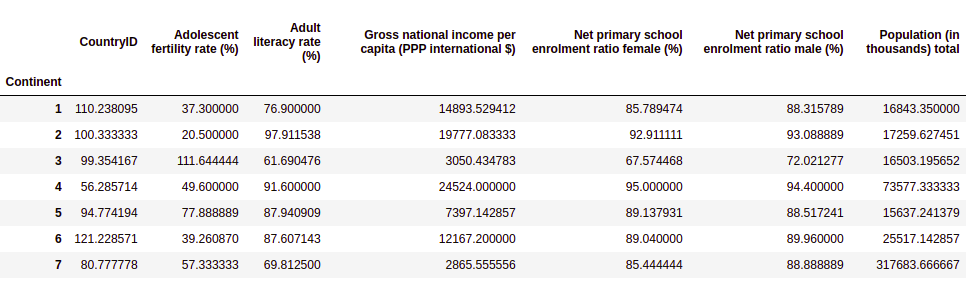Let's now group the DataFrames based on literacy rates as well:

`# Group By DataFrame on the basis of continent and select adult literacy rate(%)df.groupby('Continent').mean()['Adult literacy rate (%)']`

This results in the...

# Working with missing values

Most real-world datasets are messy and noisy. Due to their messiness and noise, lots of values are either faulty or missing. pandas offers lots of built-in functions to deal with missing values in DataFrames:

• Check missing values in a DataFrame: pandas' isnull() function checks for the existence of null values and returns True or False, where True is for null and False is for not-null values. The sum() function will sum all the True values and returns the count of missing values. We have tried two ways to count the missing values; both show the same output:
`# Count missing values in DataFramepd.isnull(df).sum()`

The following is the second method:

`df.isnull().sum()`

This results in the following output: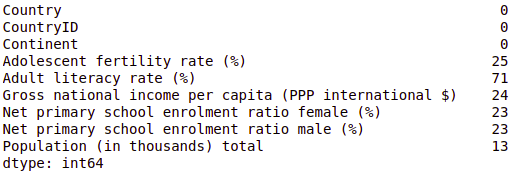• Drop missing values: A very naive approach to deal with missing values is to drop them for analysis purposes. pandas has the dropna() function to drop or delete such observations from the DataFrame. Here, the inplace=True attribute makes the changes in...

# Creating pivot tables

A pivot table is a summary table. It is the most popular concept in Excel. Most data analysts use it as a handy tool to summarize theire results. pandas offers the pivot_table() function to summarize DataFrames. A DataFrame is summarized using an aggregate function, such as mean, min, max, or sum. You can download the dataset from the following GitHub link: https://github.com/PacktPublishing/Python-Data-Analysis-Third-Edition/tree/master/Python-Data-Analysis-Third-Edition/Ch2:

`# Import pandasimport pandas as pd# Load data using read_csv()purchase = pd.read_csv("purchase.csv")# Show initial 10 recordspurchase.head(10)`

This results in the following output: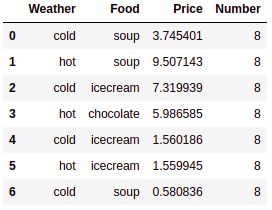In the preceding code block, we have read the purchase.csv file using the read_csv() method.

Now, we will summarize the dataframe using the following code:

`# Summarise dataframe using pivot tablepd.pivot_table(purchase,values='Number', index=['Weather',],                    columns...`

# Dealing with dates

Dealing with dates is messy and complicated. You can recall the Y2K bug, the upcoming 2038 problem, and time zones dealing with different problems. In time-series datasets, we come across dates. pandas offers date ranges, resamples time-series data, and performs date arithmetic operations.

Create a range of dates starting from January 1, 2020, lasting for 45 days, as follows:

`pd.date_range('01-01-2000', periods=45, freq='D')Output:DatetimeIndex(['2000-01-01', '2000-01-02', '2000-01-03', '2000-01-04',               '2000-01-05', '2000-01-06', '2000-01-07', '2000-01-08',               '2000-01-09', '2000-01-10', '2000-01-11', '2000-01-12',               '2000-01-13', '2000-01-14', '2000-01-15', '2000-01-16',               '2000-01-17', '2000-01-18', '2000-01-19&apos...`

# Summary

In this chapter, we have explored the NumPy and pandas libraries. Both libraries help deal with arrays and DataFrames. NumPy arrays have the capability to deal with n-dimensional arrays. We have learned about various array properties and operations. Our main focus is on data types, data type as an object, reshaping, stacking, splitting, slicing, and indexing.

We also focused on the pandas library for Python data analysis. We saw how pandas mimics the relational database table functionality. It offers functionality to query, aggregate, manipulate, and join data efficiently.

NumPy and pandas work well together as a tool and make it possible to perform basic data analysis. At this point, you might be tempted to think that pandas is all we need for data analysis. However, there is more to data analysis than meets the eye.

Having picked up the fundamentals, it's time to proceed to data analysis with the commonly used statistics functions in Chapter 3, Statistics. This includes...

# References

• Ivan Idris, NumPy Cookbook – Second Edition, Packt Publishing, 2015.
• Ivan Idris, Learning NumPy Array, Packt Publishing, 2014.
• Ivan Idris, NumPy: Beginner's Guide – Third Edition, Packt Publishing, 2015.
• L. (L.-H.) Chin and T. Dutta, NumPy Essentials, Packt Publishing, 2016.
• T. Petrou, Pandas Cookbook, Packt Publishing, 2017.
• F. Anthony, Mastering pandas, Packt Publishing, 2015.
• M. Heydt, Mastering pandas for Finance, Packt Publishing, 2015.
• T. Hauck, Data-Intensive Apps with pandas How-to, Packt Publishing, 2013.
• M. Heydt, Learning pandas, Packt Publishing, 2015.

### About the Authors

• ##### Avinash Navlani

Avinash Navlani has over 8 years of experience working in data science and AI. Currently, he is working as a senior data scientist, improving products and services for customers by using advanced analytics, deploying big data analytical tools, creating and maintaining models, and onboarding compelling new datasets. Previously, he was a university lecturer, where he trained and educated people in data science subjects such as Python for analytics, data mining, machine learning, database management, and NoSQL. Avinash has been involved in research activities in data science and has been a keynote speaker at many conferences in India.

Browse publications by this author
• ##### Armando Fandango

Dr. Armando creates AI-empowered products by leveraging reinforcement learning, deep learning, and distributed computing. Armando has provided thought leadership in diverse roles at small and large enterprises, including Accenture, Nike, Sonobi, and IBM, along with advising high-tech AI-based start-ups. Armando has authored several books, including Mastering TensorFlow, TensorFlow Machine Learning Projects, and Python Data Analysis, and has published research in international journals and presented his research at conferences. Dr. Armando’s current research and product development interests lie in the areas of reinforcement learning, deep learning, edge AI, and AI in simulated and real environments (VR/XR/AR).

Browse publications by this author
• ##### Ivan Idris

Ivan Idris has an MSc in experimental physics. His graduation thesis had a strong emphasis on applied computer science. After graduating, he worked for several companies as a Java developer, data warehouse developer, and QA analyst. His main professional interests are business intelligence, big data, and cloud computing. Ivan Idris enjoys writing clean, testable code and interesting technical articles. Ivan Idris is the author of NumPy 1.5. Beginner's Guide and NumPy Cookbook by Packt Publishing.

Browse publications by this author

### Latest Reviews

(1 reviews total)